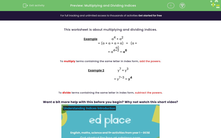# Multiply and Divide Indices

In this worksheet, students will practise multiplying and dividing indices.Key stage:  KS 3

Curriculum topic:   Number

Curriculum subtopic:   Understand Integer Powers/Real Roots

Difficulty level:#### Worksheet Overview

This activity is about multiplying and dividing indices.

Example

a4 x a2

= ( a x a x a x a) x (a x a)

= a4+2 = a6

To multiply terms containing the same letter in index form, we simply add the powers.

Example 2

y7 x y3

= y7+3

= y10

To divide terms containing the same letter in index form, we need to subtract the powers.

Example

y8 ÷ y3

= y8-3

= y5

Want a bit more help with this before you begin? Why not watch this short video?

### What is EdPlace?

We're your National Curriculum aligned online education content provider helping each child succeed in English, maths and science from year 1 to GCSE. With an EdPlace account you’ll be able to track and measure progress, helping each child achieve their best. We build confidence and attainment by personalising each child’s learning at a level that suits them.

Get started